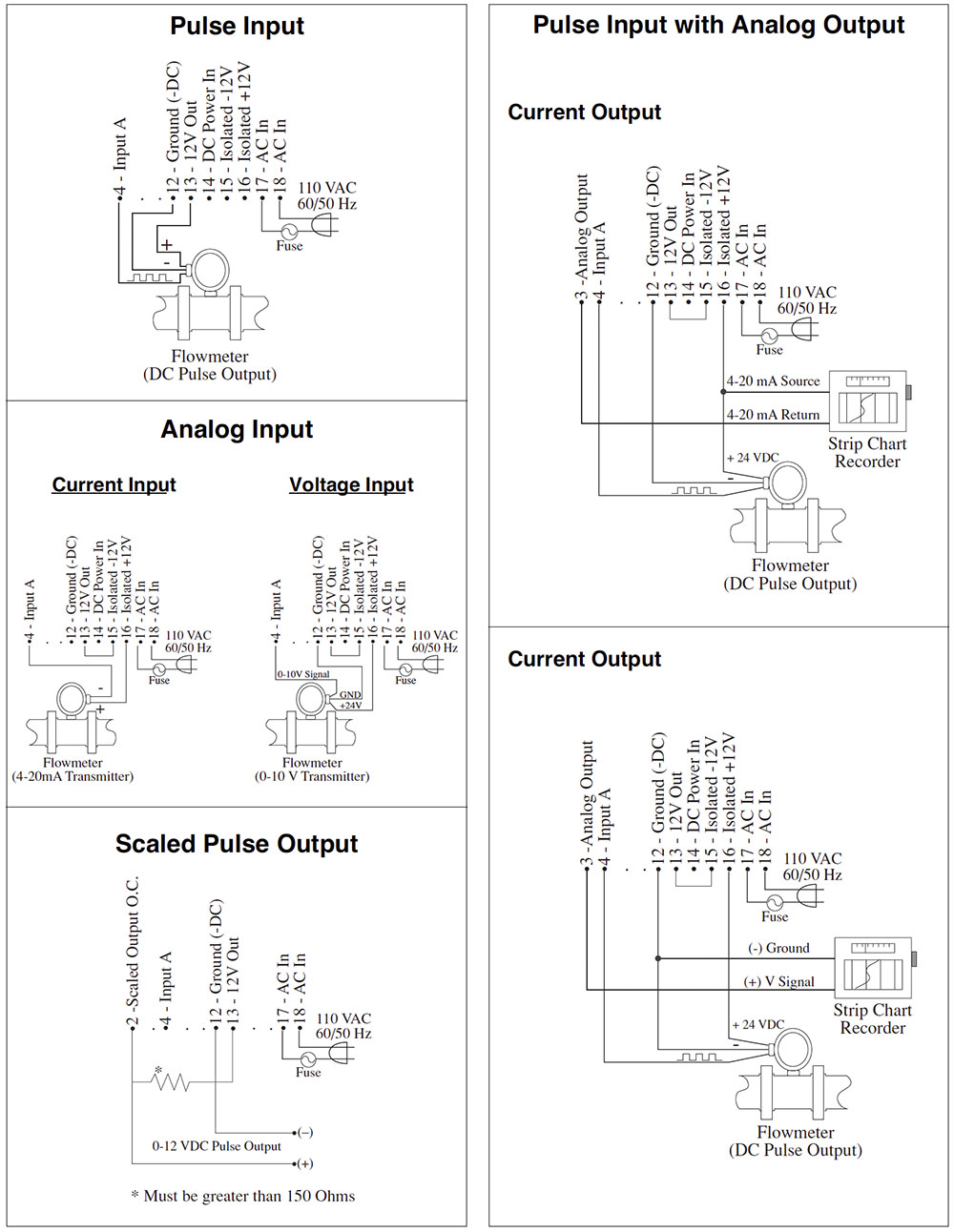# KEPtrol R/T (KRT)

8 Digit Flow Rate & Flow Totalizer

Category:

The KEPtrol R/T (KRT) ratemeter-totalizer can accept up to 20,000 pulses per second. The analog input version accepts inputs, such as 4 to 20mA or 1 to 5V. The standard KEPtrol R/T has two separate, 8 digit, floating decimal, “K” factors to convert the inputs to meaningful total and rate data. An optional 16 point K-factor can linearize flow from meter outputs. The user, with the push of a button, can toggle back and forth to view the total, the rate of flow and the grand total count. Two control outputs can be assigned independently by the user to activate at preset limit of total, rate or grand total.

## Features

• Display Total, Rate or Grand Total
• Two Alarm Outputs,
• User Selectable for Total, Rate or Grand Total
• Pulse Input to 20 kHz Count Frequency
• K-Factor Programmable to 8 Places
• Two Way RS232/422 Communications Option
• NEMA 4X/IP 65 Front Panel
• Scalable Analog Output of Rate or Total (optional)
• 16 Point Linearization Option

(Coming Soon)

## FAQ

 For Pulse Inputs the Count K-Factor is equal to the number of pulses per unit of measure which is determined by the flowmeter. (This Information is typically on the flowmeter calibration sheet or stamped on the flowmeter itself).The Rate K-Factor is calculated by dividing the Count K-Factor by the time conversion factor.Example:Count K-Factor = pulses per unit of measure (gallon, foot, revolution etc.…).Rate K-Factor (rate per second) = pulses per unit / 1Rate K-Factor (rate per minute) = pulses per unit / 60Rate K-Factor (rate per hour) = pulses per unit /3600

 Rate K- Factor: 10000/R, where R = high output rating (20mA or 5V) of transmitter. 10000 divided by 20mA or 5V rating of transmitter. Eg. 20mA rating of transmitter is 1500 gal. per min. The rate K – Factor to key into the unit for gal. per min. is 6.6666667 (10000 divided by 1500).The Count K-Factor can be calculated by modifying the Rate K-Factor to reflect the rate in units per second. If the Rate is set for rate per minute, multiply the Rate K-Factor by 60; if rate per hour, multiply the rate K-Factor by 3600.Counter K-Factor: = 6.6666667 x 60 = 400

The Factory back door code for the KEPtrol R/T can be obtained by calling the factory or send an email.

 Wiring a switch between Terminal 13 and Terminal 5 can remotely reset the unit. The Remote Reset is positive edge triggered; once it is reset by a 3 -30Vdc signal the unit will reset. If the reset is then held high the unit will continue to count until the signal is removed and applied again

Preset A and Preset B activate Relay A and Relay B at a given setpoint. When entering a value into either one of these Presets it will cause the corresponding relay to change state at that setpoint. The Relays can also be programmed to follow Rate or Total.

Yes, the KEPtrol R/T front panel is NEMA 4X rated. This rating is also comparable to the European standard IP65.

The user can view the Rate and Total of flow by toggling between the two displays with the Rate/Total button on the front of the unit. The user can also view the Grand Total by pushing the “Enter” button once while viewing the Total. The Grand Total will be the flashing Totalizer. Pushing the “Enter” button again will return you to the Total.

The KEPtrol R/T has EEPROM memory and is capable of storing program and count data for a minimum of 10 years. This is also referred to as non-volatile memory

There are two DC outputs available when the unit is powered with AC Voltage. The D.C. outputs are rated at 100ma maximum current draw

Below you will find typical wiring diagrams to help you wire a flowmeter to the KEPtrol R/TNumber of items found